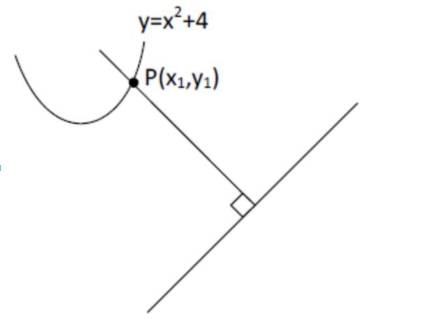# If P is a point on the parabolaQuestion:

If $P$ is a point on the parabola $y=x^{2}+4$ which is closest to the straight line $y=4 x-1$, then the co-ordinates of $\mathrm{P}$ are :

1. (1) $(-2,8)$

2. (2) $(1,5)$

3. (3) $(3,13)$

4. (4) $(2,8)$

Correct Option: , 4

Solution:

$\left.\frac{d y}{d x}\right|_{p}=4$

$\therefore 2 x_{1}=4$$\Rightarrow x_{1}=2$

$\therefore$ Point will be $(2,8)$